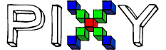Can we calculate distance to object in Arduino

Hi. Can we calculate the distance of an object defined to the camera from the camera ??
Can you help me ??
@edge @freed @Edward

Hi @Robotikman,

The forum includes a search function, which you can use to see if your question has been answered before. I searched for “calculate distance” and found the following, which should be helpful to answer your question: Determie Object Speed and Distance

Thanks,
Jesse

How can we calculate PPI on this camera ??
I don’t know if the two are the same thing.
As I understand it can be done based on these values.
If we adapt it according to the frame of the object that the camera sees. How can I calculate on this camera ??
Some objects are large, some objects are small.
This calculation should be consistent.
I want to calculate the distance for objects of all sizes.

The pixy I have is the first original version.

Keep in mind that “Pixels per Inch” is constant for a given distance from Pixy. If the objects you are interested in are a fixed distance from Pixy, you can figure out PPI and you can then figure out how big the objects are.

But if the objects are moving closer and farther away from Pixy, which is much more common, PPI isn’t going to work for you. But if (for example) the objects you are interested in are of known size, you can calculate the distance to that object based on its width.

Try this:
Take the object you’re interested in detecting, and measure its width by looking at the width that your Arduino is printing out for that object (assuming Pixy is detecting it and you’re running an example program like hello_world). This reported width is in pixels. Record both the width and the distance from the object to Pixy. Do this for several distances and plot the (width, distance) pairs on a graph. This graph can be used to then determine the distance from Pixy to the object, given its width in pixels (which Pixy provides).

Edward

Yes you are right. Based on this information, I calculated a roughly distance by calculating the area information of the frame. This works, but it’s not exactly what I’m looking for. You know that not every object is the same size. Some are very high, some are very wide. Since it differs from object to object, it creates difficulties in obtaining the desired distance information. I guess I didn’t explain it fully, but as the dimensions of the objects change, the distance information also changes. This equation works well if the objects we’re working with have the same dimensions. This is not what I’m looking for.

This only works if you know the width or height of the object… it will not work for any object of arbitrary width or height.

Edward